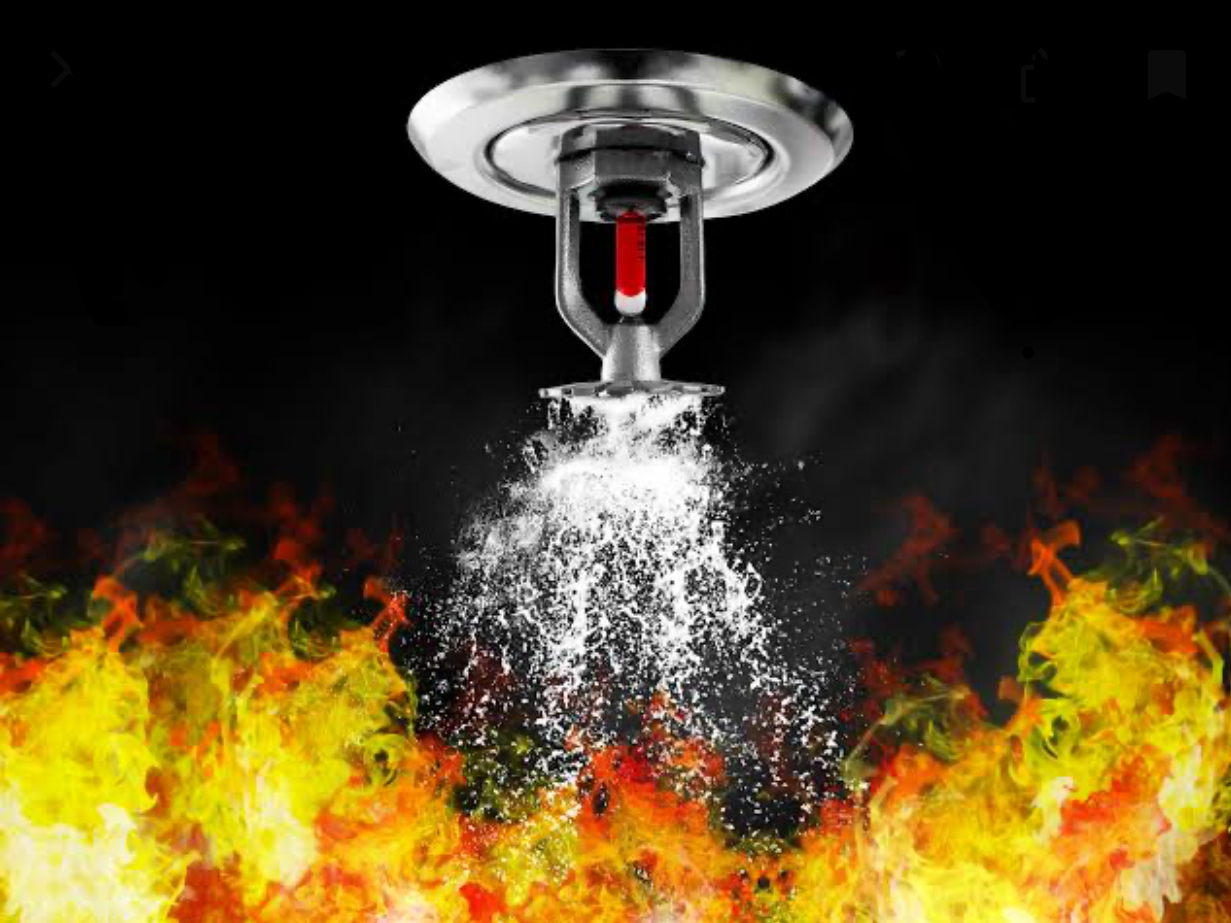# Sprinklers Distribution in water suppression system

FIRE Sprinklers Distribution in water suppression system :From NFPA tables according to hazard We get four important notes which used in our design. Maximum and Minimum spacing between two Fire Sprinkler,Maximum and Minimum spacing between sprinkler and wall.Sprinklers Distribution in water suppression system

## Sprinkler Distribution in water suppression system

1. NFPA Codes :

• Maximum space between 2 FIRE Sprinklers = 4.6m
• Minimum Space between 2  FIRE Sprinklers= 1.8m
• Maximum Space for Wall and FIRE Sprinkler= 2.3m
• Minimum Space for Wall and FIRE Sprinkler 10.2cm

### 2. Second Step :

we use the following 3 rules :
• Length / 4.6
• Width / 4.6
• follow this distribution : x 2x y 2y
• x : location between wall and Sprinkler
• 2x : location between 2 sprinklers

3. sum the values of x and y and solve for x and y such that
• sum x = Length of Zone
• sum y = Width of Zone

4. distribute distance of Fire sprinklers according to values of x and y

Example :

Room ( 10m x 5m ) and i want distribute sprinklers for it ?

### 1 . Step apply rule :

Length / 4.6 = 10/ 4.6 = 2.20 ( 3 by round)
this means i draw 3 Horizantal lines in zone relative to Length

Width/ 4.6 = 5m/ 4.6 = 1.1 ( 2 by round)
this means i draw 2 Vertical lines relative to width.

### 2. .Apply Distribution( look at figure)

3. Apply second rule :

sum x = L
sum y = W
using design we have :
x+2x+2x+x = 10
6x = 10
x= 1.6m
this means
Distance between Sprinklers and wall = 1.6m
Distance between 2 Sprinklers = 3.2m

Similarly for Y :
4y=5
y = 1.25m
this means
Distance between sprinkler and wall (vertical)  =1.25m
Distance between 2 sprinklers = 2.5m